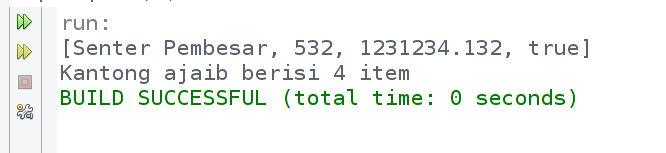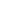| lesson material | material summary | questions and answers | definitions | types and examples | other information | materi pelajaran | ringkasan materi | pertanyaan dan jawaban | definisi | jenis-jenis dan contoh-contoh | informasi lainnya |

# Learning Java: Using Array to Store Many ThingsWhat will we do if we have a lot of data that will be stored in Vairabel?
Suppose we want to store the names of friends in a variable.
Then maybe we will do it like this:
``````String namaTeman1 = "Linda";
String namaTeman2 = "Santi";
String namaTeman3 = "Susan";
String namaTeman4 = "Mila";
String namaTeman5 = "Ayu";
``````
This is fine.
However…
The problem is when there is a lot of data, for example there are 100 data, of course tired of not making that many variables.
Therefore, we can store it all in an array.
What is an Array?
An array is a variable that can store a lot of data in one variable.The array index always starts from `0`...
... and need to know also, the index is not always in the form of numbers. It can also be characters or text.

## How to make arrays in Java

How to make an empty array:
``````// cara pertama
String[] nama;

// cara kedua
String nama[];

// cara ketiga dengan kata kunci new
String[] nama = new String;
``````
Take note :
• We use square brackets `[]`to make arrays;
• Square brackets can be placed after the data type or array name;
• Numbers `5`in parentheses means the limit or size of the array.
An empty array is ready to be filled with data. Be sure to fill it with data that matches the data type.
We can fill it like this:
``````nama = "Linda";
nama = "Santi";
nama = "Susan";
nama = "Mila";
nama = "Ayu";
``````
Or if you don't want to bother, you can create an array and fill it right away.
``````String[] nama = {"Linda", "Santi", "Susan", "Mila", "Ayu"};
``````

## Retrieving Data from Arrays

As we already know, arrays have an index to make it easier for us to access the data.
Therefore, we can retrieve the data in this way:
``````// membuat array
String[] nama = {"Linda", "Santi", "Susan", "Mila", "Ayu"};

// mengambil data array
System.out.println(teman);
``````
What do you think about the output?
Yep! really, the output is:
``````Susan
``````
Because it is `Susan`located in the 2nd index.

## Using looping

Retrieving data one by one from an array may be quite tiring, because we have to re-name the array with a different index.
Example:
``````System.out.println(teman);
System.out.println(teman);
System.out.println(teman);
System.out.println(teman);
``````
What if the array data is up to 1000, then we have to type the code a thousand times.
Therefore, this is where the repetition plays.
Pay attention :
There we use attributes `length`to take the length of the array.
So, looping will be done as much as the contents of the array.

## Now Let's Exercise

Please make a class named `Buah`, then follow the following code:
``````import java.util.Scanner;

public class Buah {
public static void main(String[] args) {

// membuat array buah-buahan
String[] buah = new String;

// membuat scanner
Scanner scan = new Scanner(System.in);

// mengisi data ke array
for( int i = 0; i < buah.length; i++ ){
System.out.print("Buah ke-" + i + ": ");
buah[i] = scan.nextLine();
}

System.out.println("---------------------------");

// menampilkan semua isi array
for( String b : buah ){
System.out.println(b);
}

}
}
``````
The output results:Pay attention :
There we use the foreach loop to display the contents of the array.
As we have learned in the Repetition material in Java , we can use this loop to display the contents of the array.

## Multi Dimensional Arrays

Multi-dimensional arrays mean arrays that have more than one dimension.
Or we can call an array in an array.
The number of dimensions is unlimited, depending on how much we can.
The following example is a two-dimensional array:
``````String[][] kontak = {
{"Lili","08111"},
{"Lala","08122"},
{"Maya","08133"}
};
``````
The index to the `0`array `kontak`contains an array `{"lili","08111"}`.Example of how to access data from a two-dimensional array:## Examples of Multi-Dimensional Array Programs

To make our understanding of multi-dimensional arrays even stronger, let's try to make a program.
Please create a new class named `RuangKelas`then follow the following code:
``````import java.util.Scanner;

public class RuangKelas {
public static void main(String[] args) {

// Membuat Array dan Scanner
String[][] meja = new String;
Scanner scan = new Scanner(System.in);

// mengisi setiap meja
for(int bar = 0; bar < meja.length; bar++){
for(int kol = 0; kol < meja[bar].length; kol++){
System.out.format("Siapa yang akan duduk di meja (%d,%d): ", bar, kol);
meja[bar][kol] = scan.nextLine();
}
}

// menampilkan isi Array
System.out.println("-------------------------");
for(int bar = 0; bar < meja.length; bar++){
for(int kol = 0; kol < meja[bar].length; kol++){
System.out.format("| %s | \t", meja[bar][kol]);
}
System.out.println("");
}
System.out.println("-------------------------");
}
}
``````
The output results:In the program, we use nesting looping to take input and display its output .
Because a two-dimensional array is similar to a table, we must repeat the rows and columns.
Then what about 3D, 4D, 5D arrays, and so on?
Of course we have to make as many nesting loops as possible.
For three, yes for three repetitions.

## Array List

The arrays that we discussed above actually have some disadvantages, such as:
• Not able to store data of different types.
• The size is not dynamic.
Therefore, there is an Array List that covers these shortcomings.
Array list is a class that allows us to create an object to hold anything.
To use the Array List, we must import it first.
``````import java.util.ArrayList;
``````
After that, we can create an Array List object like this:
`````` ArrayList al = new ArrayList();
``````
Let's try ...

### Example Program with Array List

Please create a class with a name `Doraemon`, then follow the following code:
``````import java.util.ArrayList;

public class Doraemon {
public static void main(String[] args) {

// membuat objek array list
ArrayList kantongAjaib = new ArrayList();

// Mengisi kantong ajaib dengan 5 benda

// menghapus tikus dari kantong ajaib
kantongAjaib.remove("tikus");

// Menampilkan isi kantong ajaib
System.out.println(kantongAjaib);

// menampilkan banyak isi kantong ajaib
System.out.println("Kantong ajaib berisi "+ kantongAjaib.size() +" item");
}
}
``````
The output results:Because array list ( `kantongAjaib`) is an object made from the Array List class, it has a method to do something.
• Function `add()`to add something to the Array List;
• Function `remove()`to delete something in the Array List;
• Function `size()`to retrieve the size of the Array List;
• Function `get(id)`to retrieve items in an Array List based on a particular ID or index.
• and many other functions.
##### 0 Komentar untuk "Learning Java: Using Array to Store Many Things"

Silahkan berkomentar sesuai artikel

Template By Kunci Dunia